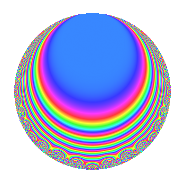# Properties

 Label 672.2.i.dLevel 672 Weight 2 Character orbit 672.i Analytic conductor 5.366 Analytic rank 0 Dimension 8 CM No Inner twists 8

# Related objects

## Newspace parameters

 Level: $$N$$ = $$672 = 2^{5} \cdot 3 \cdot 7$$ Weight: $$k$$ = $$2$$ Character orbit: $$[\chi]$$ = 672.i (of order $$2$$ and degree $$1$$)

## Newform invariants

 Self dual: No Analytic conductor: $$5.36594701583$$ Analytic rank: $$0$$ Dimension: $$8$$ Coefficient field: 8.0.3317760000.1 Coefficient ring: $$\Z[a_1, \ldots, a_{7}]$$ Coefficient ring index: $$2^{6}$$ Sato-Tate group: $\mathrm{SU}(2)[C_{2}]$

## $q$-expansion

Coefficients of the $$q$$-expansion are expressed in terms of a basis $$1,\beta_1,\ldots,\beta_{7}$$ for the coefficient ring described below. We also show the integral $$q$$-expansion of the trace form.

 $$f(q)$$ $$=$$ $$q + ( -\beta_{2} - \beta_{3} ) q^{3} -\beta_{3} q^{5} + ( -1 - \beta_{6} ) q^{7} + ( 2 + \beta_{5} ) q^{9} +O(q^{10})$$ $$q + ( -\beta_{2} - \beta_{3} ) q^{3} -\beta_{3} q^{5} + ( -1 - \beta_{6} ) q^{7} + ( 2 + \beta_{5} ) q^{9} + \beta_{4} q^{11} + ( -2 \beta_{2} - \beta_{3} ) q^{13} + ( -1 + \beta_{5} ) q^{15} + ( 2 \beta_{2} + \beta_{3} ) q^{19} + ( \beta_{1} + \beta_{2} + \beta_{3} + \beta_{4} ) q^{21} + 2 \beta_{5} q^{23} + 3 q^{25} + ( -\beta_{2} - 4 \beta_{3} ) q^{27} + 2 \beta_{4} q^{29} + 2 \beta_{6} q^{31} + ( \beta_{6} - \beta_{7} ) q^{33} + ( \beta_{3} + \beta_{4} ) q^{35} + ( 5 + \beta_{5} ) q^{39} + 2 \beta_{7} q^{41} + ( 2 \beta_{1} + \beta_{4} ) q^{43} + ( 2 \beta_{2} - \beta_{3} ) q^{45} + 2 \beta_{7} q^{47} + ( -5 + 2 \beta_{6} ) q^{49} + 2 \beta_{6} q^{55} + ( -5 - \beta_{5} ) q^{57} + 7 \beta_{3} q^{59} + ( -2 \beta_{2} - \beta_{3} ) q^{61} + ( -2 - \beta_{5} - 2 \beta_{6} - \beta_{7} ) q^{63} + 2 \beta_{5} q^{65} + ( -2 \beta_{1} - \beta_{4} ) q^{67} + ( 2 \beta_{2} - 4 \beta_{3} ) q^{69} -4 \beta_{5} q^{71} -6 \beta_{6} q^{73} + ( -3 \beta_{2} - 3 \beta_{3} ) q^{75} + ( 6 \beta_{3} - \beta_{4} ) q^{77} + 10 q^{79} + ( -1 + 4 \beta_{5} ) q^{81} -5 \beta_{3} q^{83} + ( 2 \beta_{6} - 2 \beta_{7} ) q^{87} + ( 2 \beta_{1} + 2 \beta_{2} + \beta_{3} + \beta_{4} ) q^{91} + ( -2 \beta_{1} - 2 \beta_{4} ) q^{93} -2 \beta_{5} q^{95} + 2 \beta_{6} q^{97} + ( -2 \beta_{1} + \beta_{4} ) q^{99} +O(q^{100})$$ $$\operatorname{Tr}(f)(q)$$ $$=$$ $$8q - 8q^{7} + 16q^{9} + O(q^{10})$$ $$8q - 8q^{7} + 16q^{9} - 8q^{15} + 24q^{25} + 40q^{39} - 40q^{49} - 40q^{57} - 16q^{63} + 80q^{79} - 8q^{81} + O(q^{100})$$

Basis of coefficient ring in terms of a root $$\nu$$ of $$x^{8} - 8 x^{6} + 13 x^{4} + 12 x^{2} + 36$$:

 $$\beta_{0}$$ $$=$$ $$1$$ $$\beta_{1}$$ $$=$$ $$($$$$-\nu^{6} - 10 \nu^{4} + 71 \nu^{2} - 6$$$$)/24$$ $$\beta_{2}$$ $$=$$ $$($$$$-\nu^{7} + 14 \nu^{5} - 97 \nu^{3} + 138 \nu$$$$)/144$$ $$\beta_{3}$$ $$=$$ $$($$$$-\nu^{7} + 14 \nu^{5} - 25 \nu^{3} - 78 \nu$$$$)/72$$ $$\beta_{4}$$ $$=$$ $$($$$$\nu^{6} - 6 \nu^{4} - 7 \nu^{2} + 30$$$$)/12$$ $$\beta_{5}$$ $$=$$ $$($$$$-\nu^{6} + 6 \nu^{4} - 9 \nu^{2} + 2$$$$)/8$$ $$\beta_{6}$$ $$=$$ $$($$$$\nu^{7} - 6 \nu^{5} + 5 \nu^{3} + 18 \nu$$$$)/12$$ $$\beta_{7}$$ $$=$$ $$($$$$-3 \nu^{7} + 26 \nu^{5} - 59 \nu^{3} + 54 \nu$$$$)/24$$
 $$1$$ $$=$$ $$\beta_0$$ $$\nu$$ $$=$$ $$($$$$\beta_{7} + \beta_{6} - 2 \beta_{3} - 2 \beta_{2}$$$$)/4$$ $$\nu^{2}$$ $$=$$ $$($$$$-2 \beta_{5} - 3 \beta_{4} + 8$$$$)/4$$ $$\nu^{3}$$ $$=$$ $$($$$$3 \beta_{7} + 3 \beta_{6} - 2 \beta_{3} - 14 \beta_{2}$$$$)/4$$ $$\nu^{4}$$ $$=$$ $$($$$$-8 \beta_{5} - 15 \beta_{4} - 6 \beta_{1} + 38$$$$)/4$$ $$\nu^{5}$$ $$=$$ $$($$$$15 \beta_{7} + 21 \beta_{6} + 16 \beta_{3} - 50 \beta_{2}$$$$)/4$$ $$\nu^{6}$$ $$=$$ $$($$$$-62 \beta_{5} - 63 \beta_{4} - 36 \beta_{1} + 164$$$$)/4$$ $$\nu^{7}$$ $$=$$ $$($$$$57 \beta_{7} + 141 \beta_{6} + 142 \beta_{3} - 194 \beta_{2}$$$$)/4$$

## Character Values

We give the values of $$\chi$$ on generators for $$\left(\mathbb{Z}/672\mathbb{Z}\right)^\times$$.

 $$n$$ $$127$$ $$421$$ $$449$$ $$577$$ $$\chi(n)$$ $$1$$ $$-1$$ $$-1$$ $$-1$$

## Embeddings

For each embedding $$\iota_m$$ of the coefficient field, the values $$\iota_m(a_n)$$ are shown below.

For more information on an embedded modular form you can click on its label.

Label $$\iota_m(\nu)$$ $$a_{2}$$ $$a_{3}$$ $$a_{4}$$ $$a_{5}$$ $$a_{6}$$ $$a_{7}$$ $$a_{8}$$ $$a_{9}$$ $$a_{10}$$
209.1
 −2.15988 + 0.258819i 0.578737 − 0.965926i 0.578737 + 0.965926i −2.15988 − 0.258819i 2.15988 + 0.258819i −0.578737 − 0.965926i −0.578737 + 0.965926i 2.15988 − 0.258819i
0 −1.58114 0.707107i 0 1.41421i 0 −1.00000 2.44949i 0 2.00000 + 2.23607i 0
209.2 0 −1.58114 0.707107i 0 1.41421i 0 −1.00000 + 2.44949i 0 2.00000 + 2.23607i 0
209.3 0 −1.58114 + 0.707107i 0 1.41421i 0 −1.00000 2.44949i 0 2.00000 2.23607i 0
209.4 0 −1.58114 + 0.707107i 0 1.41421i 0 −1.00000 + 2.44949i 0 2.00000 2.23607i 0
209.5 0 1.58114 0.707107i 0 1.41421i 0 −1.00000 2.44949i 0 2.00000 2.23607i 0
209.6 0 1.58114 0.707107i 0 1.41421i 0 −1.00000 + 2.44949i 0 2.00000 2.23607i 0
209.7 0 1.58114 + 0.707107i 0 1.41421i 0 −1.00000 2.44949i 0 2.00000 + 2.23607i 0
209.8 0 1.58114 + 0.707107i 0 1.41421i 0 −1.00000 + 2.44949i 0 2.00000 + 2.23607i 0
 $$n$$: e.g. 2-40 or 990-1000 Embeddings: e.g. 1-3 or 209.8 Significant digits: Format: Complex embeddings Normalized embeddings Satake parameters Satake angles

## Inner twists

Char. orbit Parity Mult. Self Twist Proved
1.a Even 1 trivial yes
3.b Odd 1 yes
7.b Odd 1 yes
8.b Even 1 yes
21.c Even 1 yes
24.h Odd 1 yes
56.h Odd 1 yes
168.i Even 1 yes

## Hecke kernels

This newform can be constructed as the intersection of the kernels of the following linear operators acting on $$S_{2}^{\mathrm{new}}(672, [\chi])$$:

 $$T_{5}^{2} + 2$$ $$T_{11}^{2} - 12$$ $$T_{13}^{2} - 10$$